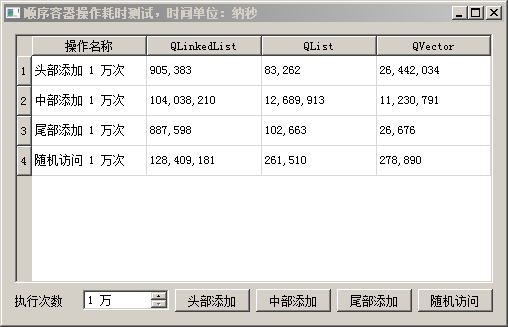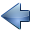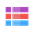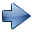9.2 顺序容器：QVector、QStack

9.2.1 向量 QVector

QVector<  QVector<int>  >  vc;
vc.resize(10);
for(int i=0; i<10; i++)
{
vc[i].resize(10);
}

（1）构造函数
QVector()    //默认构造函数
QVector(int size)    //初始化 size 个元素的向量，元素初始值为类型 T 默认构造函数生成的值
QVector(int size, const T & value)    //用 value 值初始化 size 个元素的向量
QVector(const QVector<T> & other)    //复制构造函数
QVector(QVector<T> && other)    //移动构造函数
QVector(std::initializer_list<T> args)    //初始化列表构造函数
~QVector()    //析构函数

（2）添加和分配空间函数

void    append(const T & value)      //将 value 添加到向量尾部
void    push_back(const T & value) //将 value 添加到向量尾部，STL风格
void    prepend(const T & value)    //将 value 添加到向量头部
void    push_front(const T & value)//将 value 添加到向量头部，STL风格

void    insert(int i, const T & value)
void    insert(int i, int count, const T & value)

insert() 函数支持的最大序号是 size() ，即元素总数，如果 i 等于 size() ，那么元素添加到向量末尾。如果 i >size()  ，程序会因为访问越界而崩溃。其他带序号 i 的函数，序号范围仅支持 0 ~ size()-1 ，只有 insert() 例外多支持一个添加到末尾。

QVector<T> &    fill(const T & value, int size = -1)

int    count() const
int    size() const
int    length() const

void    resize(int size)

resize() 函数会直接影响向量里的元素个数，如果希望在不影响元素个数的情况下扩容，那么也有相应的函数。下面三个函数就是用于设置、获取容量，但不影响元素个数：
void    reserve(int size)  //为 size 个元素分配空间，但是不增加任何新元素，向量的元素总数不变
int    capacity() const    //查询向量内部的存储容量，容量一般大于等于 count()
void    squeeze()           //释放不需要的额外容量，与 reserve()  函数功能相反

capacity() 用于查询向量的存储空间，一般大于等于 count() 。
squeeze() 用于释放额外空间，与 reserve(  size  ) 的操作正好相反。

resize( size ) 直接影响元素个数，如果参数里数值比旧的尺寸大，那么扩容并添加新元素，向量元素总数变为参数指定的个数；

QVector<int> va = {1, 2, 3};
va.reserve(10);
va = 1;    //！！！错误示范
reserve(10) 会为向量扩容，但没有新增元素，上面最后一句  va = 1 是数组越界，会导致程序崩溃。

（3）移除和删除函数

T    takeAt(int i) //卸下序号 i 位置元素，并返回该元素
T    takeFirst()    //卸下向量的头部元素，并返回该元素
T    takeLast()    //卸下向量的尾部元素，并返回该元素

void    remove(int i)     //删除序号 i 位置元素
void    removeAt(int i) //删除序号 i 位置元素
void    remove(int i, int count) //从序号 i 位置开始删除参数里 count 个数的元素
void    removeFirst()  //删除头部元素
void    pop_front()    //删除头部元素，STL风格
void    removeLast() //删除尾部元素
void    pop_back()    //删除尾部元素，STL风格

bool    removeOne(const T & t)    //如果向量里存在等于 t 的元素就删除并返回 true，否则找不到返回 false
int    removeAll(const T & t)    //删除向量中所有等于 t 的元素，并返回删除的数量
removeOne( t ) 和 removeAll( t ) 函数需要元素类型 T 支持双等号比较函数 operator==() 。

void    clear()

（4）访问和查询函数

const T &    at(int i) const    //返回序号 i 元素的常量引用，效率最高，但是不做越界判断！
T    value(int i) const
T    value(int i, const T & defaultValue) const
at( i ) 的函数效率最高，但是它不检查数组越界，一旦越界程序就会崩溃，对于该函数必须确保 0 <= i < size() 。

T &    first()    //头部元素的读写引用
const T &    first() const    //头部元素的只读引 用
T &    front()  //头部元素的读写引用，STL风格
const_reference    front() const   //头部元素的只读引用，STL风格
T &    last()    //尾部元素的读写引用
const T &    last() const    //尾部元素的只读引用
reference    back()    //尾部元素的读写引用，STL风格
const_reference    back() const    //尾部元素的只读引用，STL风格
STL风格函数返回值的 reference 是类型定义，等同 T &  ； const_reference 也是类型定义，等同 const T &  。

bool    empty() const    //判断向量是否为空，STL风格
bool    isEmpty() const //判断向量是否为空

bool    contains(const T & value) const    //查询向量是否包含等于 value 数值的元素，有就返回 true，没有就返回 false
int    count(const T & value) const    //查询向量包含几个等于 value 数值的元素，有就返回个数，没有就返回 0

int    indexOf(const T & value, int from = 0) const    //从前到后查询，从 from 序号开始查找等于 value 的元素序号，找不到时返回 -1
int    lastIndexOf(const T & value, int from = -1) const //从后向前查询，从 from 序号开始倒着查找等于 value 的元素序号，找不到就返回 -1
indexOf() 是按照序号从小到大查找，lastIndexOf() 是反过来，按照序号从大到小来查找，这两个函数如果找不到匹配的元素，就会返回 -1，要注意返回值的判断。

bool    startsWith(const T & value) const    //查询头部元素是否等于 value
bool    endsWith(const T & value) const    //查询尾部元素是否等于 value

T *    data()    //获取向量内部存储空间的读写指针，可以修改元素值
const T *    data() const    //获取向量内部存储空间的只读指针
const T *    constData() const //获取向量内部存储空间的只读指针
data() 和 constData() 返回值就是向量里真实的数组指针，如果读者希望自己直接用指针操作各个元素，就可以用这三个函数。

（5）获取子序列、替换和交换函数

QVector<T>    mid(int pos, int length = -1) const  //从序号 pos 开始复制 length 个数的元素到新向量，返回新向量
mid() 函数专门提取子序列向量，从 pos 开始复制 length 个数的元素到新向量里，返回新向量。如果 length 为 -1 或超出原向量的界限，那么从 pos 开始的所有元素都会复制到新向量里。

void    replace(int i, const T & value)

void    swap(QVector<T> & other)
swap() 函数执行效率非常高，并且不会失败。

（6）运算符函数

QVector<int> va = {1, 2, 3};
QVector<int> vb = {4, 5, 6};
QVector<int> vc;

 运算符函数 举 例 描述 bool operator!=(const QVector & other) const va != vb; va 和 vb 两个向量有元素不同，结果为 true。 QVector operator+(const QVector & other) const vc = va + vb; va 和 vb 复制拼接后生成新向量，赋值给 vc。 QVector & operator+=(const QVector & other) va += vb; 复制 vb 所有元素追加到 va 末尾。 QVector & operator+=(const T & value) va += 100 ; 添加一个元素 100 到 va 末尾。 QVector & operator<<(const QVector & other) va << vb; 复制 vb 所有元素追加到 va 末尾。 QVector & operator<<(const T & value) va << 100; 添加一个元素 100 到 va 末尾。 QVector & operator=(const QVector & other) vc = va; va 所有元素都复制一份给 vc，va本身不变。二者相等。 QVector operator=(QVector && other) //移动赋值 vc = std::move(va) ; va 所有元素都移动给 vc， va本身被清空。 bool operator==(const QVector & other) const va == vb; va 和 vb 有元素不同，结果为 false。 只有两个向量的所有元素相等并且顺序一样，它们才能算相等。 T & operator[](int i) va = 100; 获取序号为 i 的元素的读写引用，可修改向量元素。 const T & operator[](int i) const qDebug()<

（7）迭代器函数

typedef    iterator    //读写迭代器，STL风格
typedef    Iterator   //读写迭代器，Qt 风格
typedef    const_iterator    //只读迭代器，STL风格
typedef    ConstIterator    //只读迭代器，Qt风格

QVector<int> va = {1, 2, 3};
QVector<int>::iterator  it = va.begin();
while(  it != va.end() )
{
qDebug()<< (*it);
it++;   //下一个
}

iterator    begin()    //指向头部的读写迭代器
const_iterator    begin() const            //指向头部的只读迭代器
const_iterator    cbegin() const          //指向头部的只读迭代器
const_iterator    constBegin() const   //指向头部的只读迭代器，Qt 风格
iterator    end()    //指向尾部后面假想元素的读写迭代器
const_iterator    end() const            //指向尾部后面假想元素的只读迭代器
const_iterator    cend() const          //指向尾部后面假想元素的只读迭代器
const_iterator    constEnd() const   //指向尾部后面假想元素的只读迭代器，Qt风格

iterator    insert(iterator before, const T & value)    //在 before 指向的元素前面插入元素 value
iterator    insert(iterator before, int count, const T & value) //在  before 指向的元素前面插入 count 个数的 value 元素
insert() 迭代器函数返回值就会自动指向新插入的元素，如果插入多个元素，就指向新插入的第一个元素，参数里迭代器 before 不能再使用。一定要使用返回值里的迭代器进行后续遍历操作。

iterator    erase(iterator pos)    //删除 pos 指向的一个元素，返回值指向下一个元素，可能指向尾部后面假想元素
iterator    erase(iterator begin, iterator end) //删除从参数 begin 到参数 end 的元素，但是参数 end 元素不删除，总是返回参数 end

（8）容器类型转换函数

QList<T>    toList() const    //转为 Qt 列表
std::vector<T>    toStdVector() const    //转为标准库的向量

QVector<T>    fromList(const QList<T> & list)    //将参数里的列表转为向量，返回值是新向量
QVector<T>    fromStdVector(const std::vector<T> & vector)    //将参数里的标准库向量转为 Qt 向量

（9）其他内容

QDataStream &    operator<<(QDataStream & out, const QVector<T> & vector)  //输出
QDataStream &    operator>>(QDataStream & in, QVector<T> & vector)    //输入

void qSort(Container & container)  //排序
void qStableSort(Container & container)  //稳定排序

bool  operator< ( const T &t1, const T &t2 )

① 必须是可赋值类型，需要默认构造函数、复制构造函数、赋值函数 operator=() ；
② 如果希望支持查询函数，需要双等号函数 operator==()；
③ 如果希望支持排序函数，需要全局小于号函数 operator< (  const T &t1, const T &t2 ) ；
④ 如果希望支持 QDataStream 数据流输入输出，那么添加友元函数  operator<<() 和 operator>>() 。

https://qtguide.ustclug.org/QtProjects/ch09/cityposition/position.txt
position.txt 保存了全国各省市的经纬度，里面的内容举例如下：

①项目名称 cityposition，创建路径 D:\QtProjects\ch09，点击下一步；
②套件选择里面选择全部套件，点击下一步；
③基类选择 QWidget，点击下一步；
④项目管理不修改，点击完成。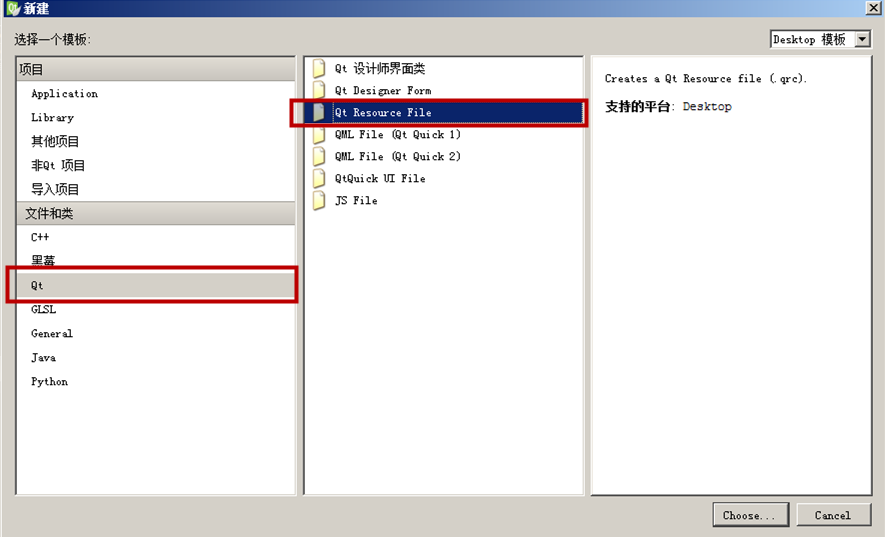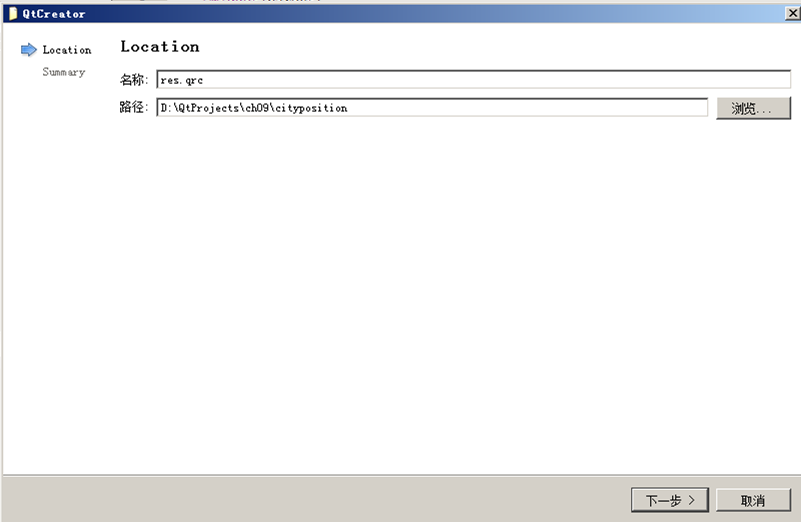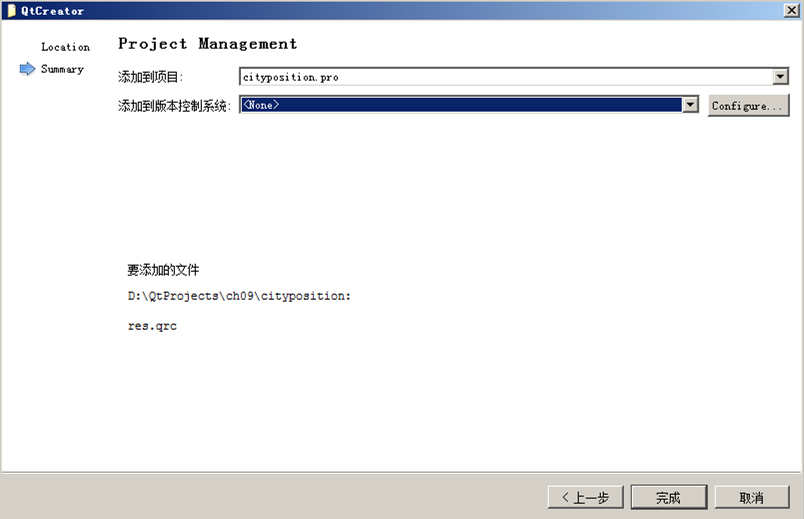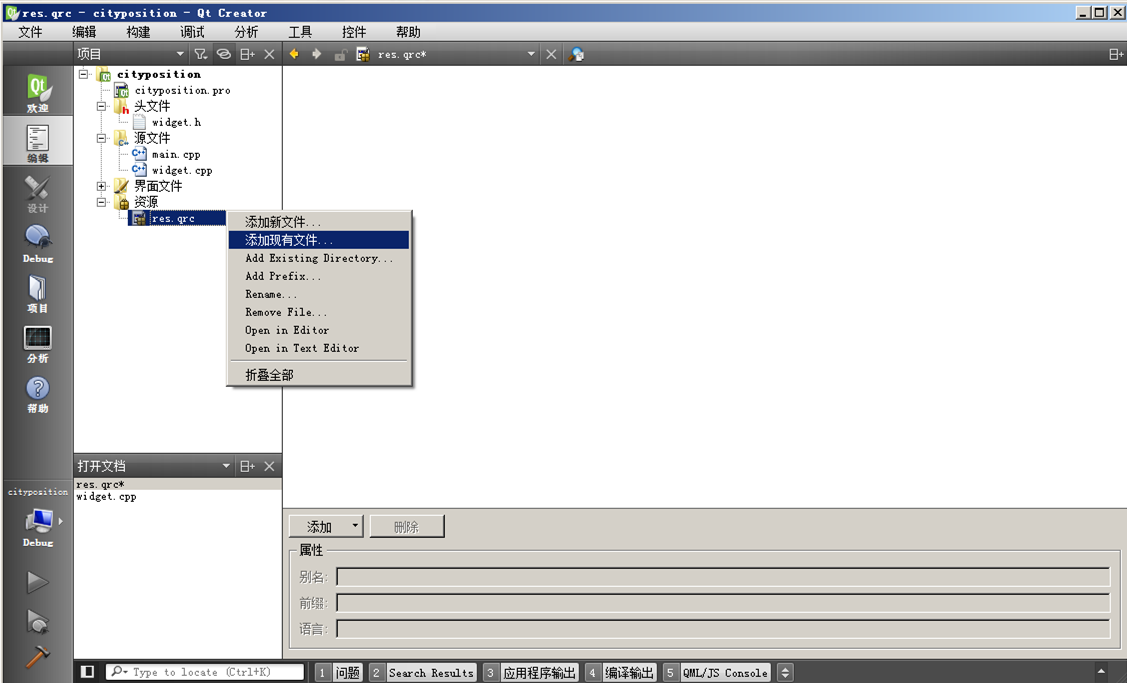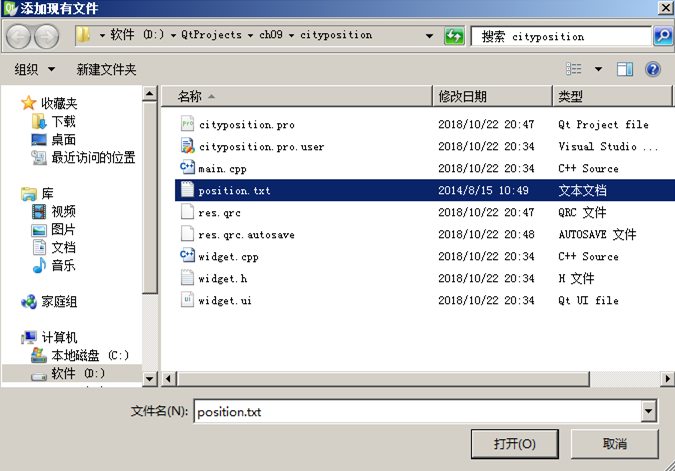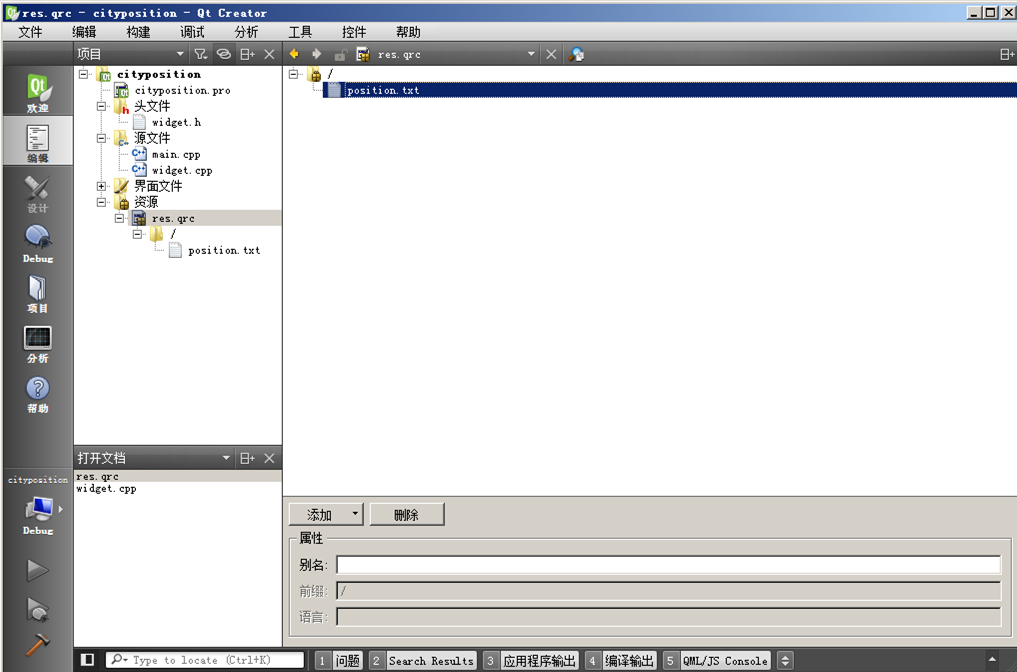:/position.txt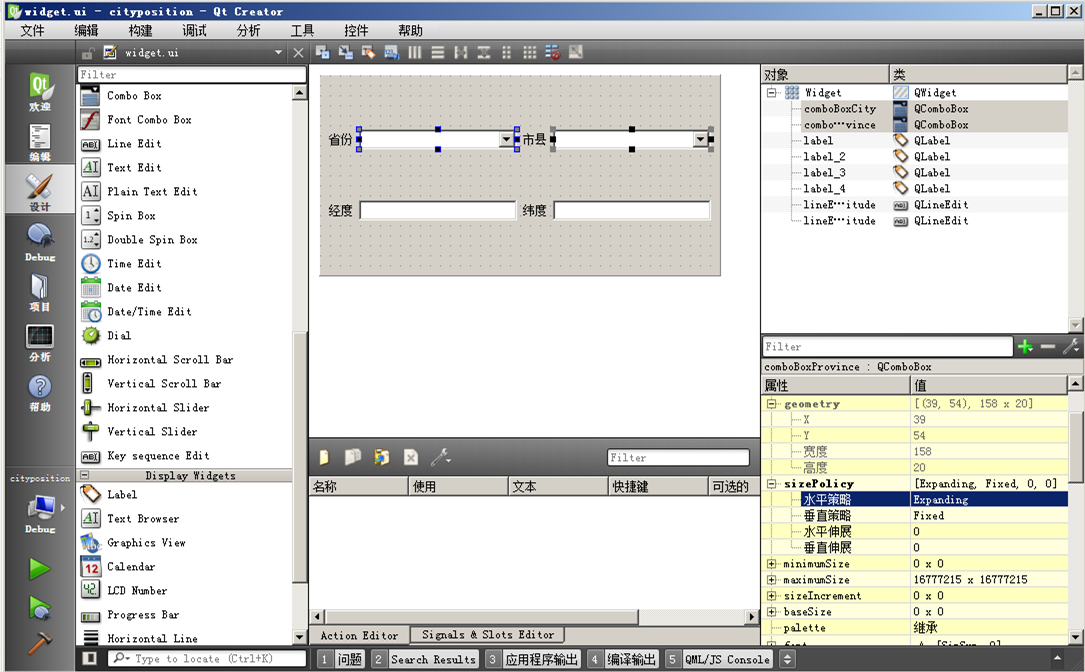#ifndef WIDGET_H
`#define WIDGET_H`

`#include <QWidget>`
`#include <QVector>`

`namespace Ui {`
`class Widget;`
`}`

`class Widget : public QWidget`
`{`
`    Q_OBJECT`

`public:`
`    explicit Widget(QWidget *parent = 0);`
`    ~Widget();`

`private slots:`
`    //省份变化时，自动加载该省的市县`
`    void onProvinceIndexChange(int index);`
`    //市县变化时，自动显示市县的经纬度到编辑框`
`    void onCityIndexChange(int index);`

`private:`
`    Ui::Widget *ui;`
`    //保存省份名称`
`    QVector<QString> m_vProvinces;`
`    //二维向量保存市县名称`
`    QVector<  QVector<QString>  > m_vCities;`
`    //二维向量保存市县的经度`
`    QVector<  QVector<double>  > m_vLongitudes;`
`    //二维向量保存市县的纬度`
`    QVector<  QVector<double>  > m_vLatitudes;`
`    //从 position.txt 加载各省市及其经纬度`
`    void loadPositions();`
`};`

`#endif // WIDGET_H`

`#include "widget.h"`
`#include "ui_widget.h"`
`#include <QDebug>`
`#include <QFile>    //文件`
`#include <QTextStream>//文本流`

`Widget::Widget(QWidget *parent) :`
`    QWidget(parent),`
`    ui(new Ui::Widget)`
`{`
`    ui->setupUi(this);`
`    //设置编辑框为只读`
`    ui->lineEditLongitude->setReadOnly(true);`
`    ui->lineEditLatitude->setReadOnly(true);`
`    //从资源文件 position.txt 读取省市经纬度`
`    loadPositions();`
`    //关联信号，省份组合框序号变化`
`    connect(ui->comboBoxProvince, SIGNAL(currentIndexChanged(int)),`
`            this, SLOT(onProvinceIndexChange(int)) );`
`    //市县组合框序号变化`
`    connect(ui->comboBoxCity, SIGNAL(currentIndexChanged(int)),`
`            this, SLOT(onCityIndexChange(int)) );`
`}`

`Widget::~Widget()`
`{`
`    delete ui;`
`}`

`//从资源文件 position.txt 读取省市经纬度`
`void Widget::loadPositions()`
`{`
`    //打开资源里的文件`
`    QFile fileIn( ":/position.txt" );`
`    //资源文件打开一般不需要判断，只要文件名对就可以打开`
`    fileIn.open( QIODevice::ReadOnly );`
`    //定义文本流`
`    QTextStream tsIn(&fileIn);`
`    tsIn.setCodec( "UTF-8" ); //该文件使用 UTF-8 文本编码`

`    //为二维向量的第一维省份添加元素空间，这样后面才能使用中括号访问`
`    m_vCities.resize( 40 );//全国省份目前只有34个，分配 40 保证够用`
`    m_vLongitudes.resize( 40 );`
`    m_vLatitudes.resize( 40 );`

`    //定义变量`
`    QString strProvBefore;//保存前一行记录的省份，用于判断是否应该添加新省份了`
`    QString strProvCur;   //当前行加载的省份`
`    QString strCity;    //当前行加载的市县`
`    int nLonD;  //经度的度`
`    int nLonM;  //经度的分`
`    int nLatD;  //纬度的度`
`    int nLatM;  //纬度的分`
`    //记录当前省份的序号`
`    int nProvIndex = -1;`
`    //开始读取，文本流不结束就一直读`
`    while( !tsIn.atEnd() )`
`    {`
`        //依次读取省份、市县、纬度的度和分、经度的度和分`
`        tsIn>>strProvCur>>strCity`
`            >>nLatD>>nLatM`
`            >>nLonD>>nLonM ;`
`        //如果当前省份不等于前一个省份，需要新增省份`
`        if( strProvBefore != strProvCur )`
`        {`
`            nProvIndex++; //省份序号增加`
`            strProvBefore = strProvCur; //保存省份，用于下一行记录判断`
`            //添加新的省份`
`            m_vProvinces<<strProvCur; //一维向量不需要提前分配，自动分配空间并追加元素`
`        }`
`        //无论省份新旧，都要添加市县、经度、纬度`
`        //添加市县`
`        m_vCities[nProvIndex] << strCity;`
`        //添加纬度，转为浮点数的度`
`        m_vLatitudes[nProvIndex] << nLatD + (double)nLatM/60.0;`
`        //添加经度`
`        m_vLongitudes[nProvIndex] << nLonD + (double)nLonM/60.0;`
`    }`
`    //从文件加载完毕`
`    //省份组合框的内容是固定的，直接添加`
`    for(int i=0; i<m_vProvinces.count(); i++)`
`    {`
`        ui->comboBoxProvince->addItem( m_vProvinces[i] );`
`    }`
`    //设置省份序号`
`    ui->comboBoxProvince->setCurrentIndex( 0 );`
`    //手动调用省份变化槽函数，显示第一个省份的所有市县到组合框`
`    onProvinceIndexChange( 0 );`
`    //手动调用市县变化槽函数，显示经纬度`
`    onCityIndexChange( 0 );`
`    //打印省份计数`
`    qDebug()<<tr("加载完毕，省份计数： %1").arg( m_vProvinces.count() );`
`}`

如果不一样就将省份序号加一，保存当前行的省份给 strProvBefore 用于下轮循环判断，将新省份名称添加到向量 m_vProvinces。

当文件所有内容读取完毕时，结束循环。

`//根据省份序号变化，自动加载该省的市县`
`void Widget::onProvinceIndexChange(int index)`
`{`
`    if(index < 0)   return; //序号不合法，不处理`
`    //清空市县组合框`
`    ui->comboBoxCity->clear();`
`    //根据省份序号，加载该省的市县到市县组合框`
`    //该省的市县计数`
`    int nCount = m_vCities[index].count();`
`    for(int i=0; i<nCount; i++)`
`    {`
`        //第一维是省序号，第二维是市县序号`
`        ui->comboBoxCity->addItem( m_vCities[index][i] );`
`    }`
`    //设置默认市县的序号`
`    ui->comboBoxCity->setCurrentIndex( 0 );`
`}`

`//根据市县序号变化，自动显示市县的经纬度到编辑框`
`void Widget::onCityIndexChange(int index)`
`{`
`    if(index < 0)   return; //序号不合法，不处理`
`    //获取省份序号，为第一个纬度`
`    int nProvIndex = ui->comboBoxProvince->currentIndex();`
`    //设置经度编辑框`
`    ui->lineEditLongitude->setText(`
`                tr("%1").arg( m_vLongitudes[nProvIndex][index] ) );`
`    //设置纬度编辑框`
`    ui->lineEditLatitude->setText(`
`                tr("%1").arg( m_vLatitudes[nProvIndex][index] ) );`
`}`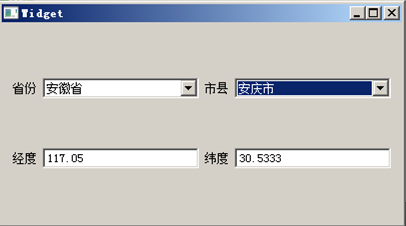9.2.2 栈 QStack

QStack 是向量 QVector 的派生类，继承了基类全部的功能，仅仅添加少许的栈操作函数。首先是进栈、出栈的函数：
void push(const T & t) //将 t 添加到栈顶
T  pop()    //取出栈顶元素并返回

T &  top()  //获取栈顶元素的读写引用
const T &    top() const  //获取栈顶元素的只读引用

void swap(QStack<T> & other)

https://www.cnblogs.com/jeavenwong/p/8176808.html

①项目名称 stacktraversal，创建路径 D:\QtProjects\ch09，点击下一步；
②套件选择里面选择全部套件，点击下一步；
③基类选择 QWidget，点击下一步；
④项目管理不修改，点击完成。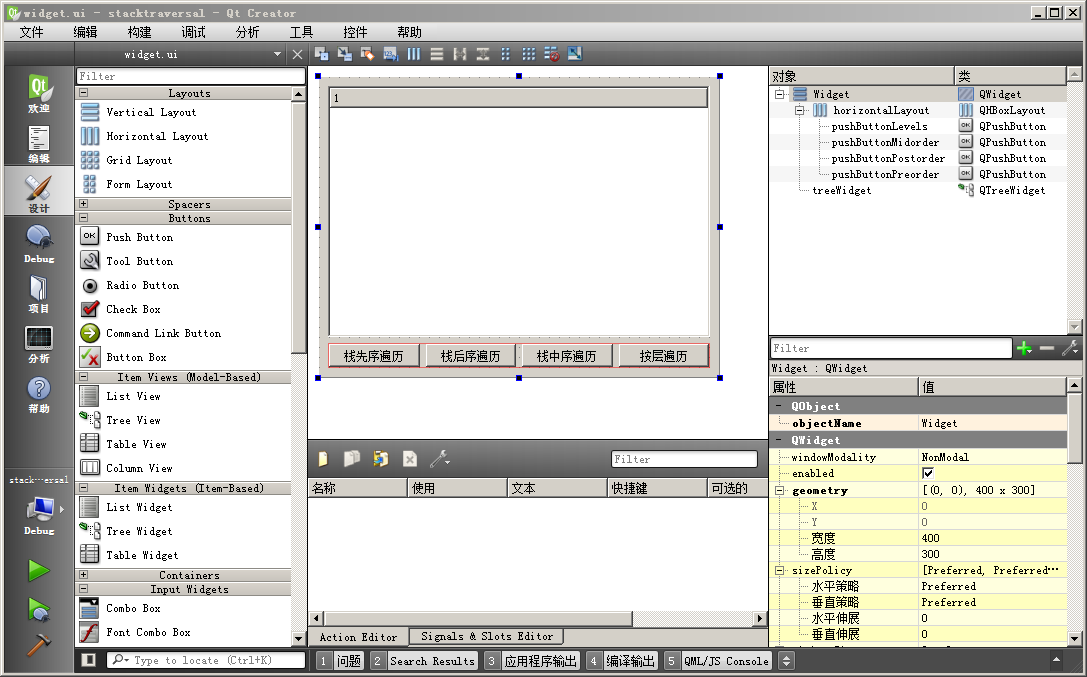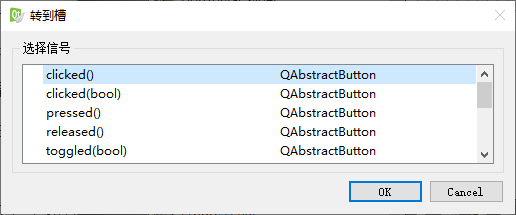#ifndef WIDGET_H
`#define WIDGET_H`

`#include <QWidget>`
`#include <QTreeWidgetItem>//树形条目`
`#include <QTreeWidget>  //树形控件`
`#include <QStack>//栈`
`#include <QQueue>//队列`

`namespace Ui {`
`class Widget;`
`}`

`class Widget : public QWidget`
`{`
`    Q_OBJECT`

`public:`
`    explicit Widget(QWidget *parent = 0);`
`    ~Widget();`

`private slots:`
`    void on_pushButtonPreorder_clicked();`

`    void on_pushButtonPostorder_clicked();`

`    void on_pushButtonMidorder_clicked();`

`    void on_pushButtonLevels_clicked();`

`private:`
`    Ui::Widget *ui;`
`    //先序遍历函数`
`    void PreorderByStack(QTreeWidgetItem *curItem);`
`    //后序遍历`
`    void PostorderByStack(QTreeWidgetItem *curItem);`
`    //中序遍历`
`    void MidorderByStack(QTreeWidgetItem *curItem);`
`    //按层遍历，队列实现`
`    void LevelsByQueue(QTreeWidgetItem *curItem, int curLevel);`
`};`

`#endif // WIDGET_H`

`#include "widget.h"`
`#include "ui_widget.h"`
`#include <QDebug>`

`Widget::Widget(QWidget *parent) :`
`    QWidget(parent),`
`    ui(new Ui::Widget)`
`{`
`    ui->setupUi(this);`
`    //树形控件只有一列数据`
`    ui->treeWidget->setColumnCount(1);`
`    //添加顶级节点`
`    QTreeWidgetItem *itemA = new QTreeWidgetItem();`
`    itemA->setText(0, "A");`
`    ui->treeWidget->addTopLevelItem( itemA );`
`    //A的两个子节点B、C`
`    QTreeWidgetItem *itemB = new QTreeWidgetItem(itemA);`
`    itemB->setText(0, "B");`
`    QTreeWidgetItem *itemC = new QTreeWidgetItem(itemA);`
`    itemC->setText(0, "C");`
`    //B的两个子节点D、E`
`    QTreeWidgetItem *itemD = new QTreeWidgetItem(itemB);`
`    itemD->setText(0, "D");`
`    QTreeWidgetItem *itemE = new QTreeWidgetItem(itemB);`
`    itemE->setText(0, "E");`
`    //C的两个子节点F、G`
`    QTreeWidgetItem *itemF = new QTreeWidgetItem(itemC);`
`    itemF->setText(0, "F");`
`    QTreeWidgetItem *itemG = new QTreeWidgetItem(itemC);`
`    itemG->setText(0, "G");`
`    //展开所有`
`    ui->treeWidget->expandAll();`
`}`

`Widget::~Widget()`
`{`
`    delete ui;`
`}`

`void Widget::on_pushButtonPreorder_clicked()`
`{`
`    //获取A节点`
`    QTreeWidgetItem *itemA = ui->treeWidget->topLevelItem(0);`
`    //进行先序遍历`
`    qDebug()<<tr("先序遍历：");`
`    PreorderByStack( itemA );`
`}`

`//使用栈实现先序遍历`
`void Widget::PreorderByStack(QTreeWidgetItem *curItem)`
`{`
`    //判断指针`
`    if(NULL == curItem) return; //空指针不处理`
`    //定义栈`
`    QStack<QTreeWidgetItem *> stackItems;`
`    //初始节点入栈`
`    stackItems.push( curItem );`
`    //用于遍历的条目变量`
`    QTreeWidgetItem *itemTreversal = NULL;`
`    while( ! stackItems.isEmpty() )`
`    {`
`        //栈顶的出栈`
`        itemTreversal = stackItems.pop();`
`        //先序是添加子节点之前部署工作代码，打印文本`
`        qDebug()<<itemTreversal->text(0);`
`        //子节点计数`
`        int nChildCount = itemTreversal->childCount();`
`        //如果是叶子就进行下一轮`
`        if( nChildCount < 1 )`
`        {`
`            continue;`
`        }`
`        else//有子节点，子节点从右往左，倒序压栈`
`        {`
`            //倒序压栈，左边子节点处于栈顶，出栈时优先处理左边子节点`
`            for(int i=nChildCount-1; i>=0; i--)`
`            {`
`                stackItems.push( itemTreversal->child(i) );`
`            }`
`        }`
`        //下一轮循环`
`    }//栈不空就一直循环处理`
`    return; //结束`
`}`

取出栈顶元素，存到 itemTreversal ；
打印 itemTreversal 的文本，即工作代码；
获取 itemTreversal 子节点计数；
如果是叶子节点就跳过后面代码，继续下轮循环；
如果不是叶子，itemTreversal  存在子节点，那么将它的子节点从右到左压入栈中，这样等会出栈时，就可以先处理左子树；

`void Widget::on_pushButtonPostorder_clicked()`
`{`
`    //获取A节点`
`    QTreeWidgetItem *itemA = ui->treeWidget->topLevelItem(0);`
`    //后序遍历`
`    qDebug()<<tr("后序遍历：");`
`    PostorderByStack( itemA );`
`}`

`//用栈实现后序遍历`
`void Widget::PostorderByStack(QTreeWidgetItem *curItem)`
`{`
`    //判断指针`
`    if(NULL == curItem) return; //空指针不处理`
`    //定义栈`
`    QStack<QTreeWidgetItem *> stackItems;`
`    //初始节点入栈`
`    stackItems.push( curItem );`
`    //遍历使用的条目变量`
`    QTreeWidgetItem *itemTraversal = NULL;`
`    QTreeWidgetItem *itemLast = NULL;   //上次弹出的节点`
`    //后序遍历时，第一步进行层次推进，父子节点先后进栈`
`    //第二步出栈回归上层，子节点先出栈打印，父节点后出栈`
`    while( ! stackItems.isEmpty() )`
`    {`
`        //后序遍历先打印所有子树，根节点要一直存在栈里，直到子树打印完才出栈`
`        itemTraversal = stackItems.top();`
`        //子节点计数`
`        int nChildCount = itemTraversal->childCount();`
`        //如果是叶子节点，推进层次截止`
`        if( nChildCount < 1 )`
`        {`
`            //出栈打印`
`            itemTraversal = stackItems.pop();`
`            qDebug()<<itemTraversal->text(0);`
`            //保存上次弹出节点，用于判断是推进层次还是回归顶层`
`            itemLast = itemTraversal;`
`            continue; //下一轮`
`        }`
`        //存在子节点时，可能是层次推进过程，也可能是回归上层的过程`
`        //如果上次出栈打印的是当前节点的最右边儿子，那么子树打印过了，就是回归上层`
`        //如果上次出栈打印的不是最右边儿子节点，那么需要进一步推进层次`
`        if( itemTraversal->child( nChildCount-1 ) != itemLast )`
`        {`
`            //深入递推，那么将子节点压栈`
`            //存在子节点，把子节点都入栈，从右向左压栈`
`            for(int i=nChildCount-1; i>=0; i--)`
`            {`
`                //左子节点优先在栈顶，优先打印左边的`
`                stackItems.push( itemTraversal->child(i) );`
`            }`
`        }`
`        else//回归上层，那么将当前节点出栈并打印`
`        {`
`            //出栈打印`
`            itemTraversal = stackItems.pop();`
`            qDebug()<<itemTraversal->text(0);`
`            //保存上次弹出节点`
`            itemLast = itemTraversal;`
`        }`
`        //下一轮`
`    }`
`    return; //完成`
`}`

获取栈顶元素存到 itemTraversal，但是栈顶元素不出栈，因为子树的根节点要等子孙遍历完才能出栈；
获取 itemTraversal 的子节点数 nChildCount；
判断 itemTraversal 如果为叶子节点，那么进入工作代码：
将栈顶元素出栈，打印节点文本，将出栈的元素存到 itemLast 供下轮循环使用，然后 continue进入下轮循环；
接着是对有子节点的 itemTraversal  进行判断，如果上一轮的 itemLast 不是 itemTraversal  最右儿子节点，那么进入推进层次的代码：
将 itemTraversal  所有子节点，按照从右到左顺序压入栈中，然后自动进入下轮循环；
如果上一轮的 itemLast 正好是 itemTraversal  最右儿子节点，那么进入回归上层的代码：
将栈顶元素出栈，打印文本，将出栈的元素存到 itemLast 供下轮循环使用，然后自动进入下轮循环。

`void Widget::on_pushButtonMidorder_clicked()`
`{`
`    //获取A节点`
`    QTreeWidgetItem *itemA = ui->treeWidget->topLevelItem(0);`
`    //中序遍历`
`    qDebug()<<tr("中序遍历：");`
`    MidorderByStack( itemA );`
`}`

`//用栈实现中序遍历`
`void Widget::MidorderByStack(QTreeWidgetItem *curItem)`
`{`
`    //判断指针`
`    if(NULL == curItem) return; //空指针不处理`
`    //定义栈`
`    QStack<QTreeWidgetItem *> stackItems;`
`    //初始节点入栈`
`    stackItems.push(curItem);`
`    //遍历的节点遍历`
`    QTreeWidgetItem *itemTraversal = NULL;`
`    //循环`
`    while( ! stackItems.isEmpty() )`
`    {`
`        //把所有层的最左节点压栈`
`        while( stackItems.top()->childCount() >= 1 )`
`        {`
`            stackItems.push( stackItems.top()->child(0) );`
`        }`
`        //总是从最左边节点开始出栈`
`        while( ! stackItems.isEmpty() )`
`        {`
`            //出栈并打印`
`            itemTraversal = stackItems.pop();`
`            qDebug()<<itemTraversal->text( 0 );`
`            //子节点计数`
`            int nChildCount = itemTraversal->childCount();`
`            //如果存在右边的节点`
`            if( nChildCount >= 2 )`
`            {`
`                //右边的子节点都入栈，压栈时从右往左，出栈时就是从左到右`
`                for(int i=nChildCount-1; i>=1; i--)`
`                {`
`                    stackItems.push( itemTraversal->child(i) );`
`                }`
`                //该节点还存在右边的子树，跳出内层循环，对右边子树按照外层循环操作`
`                break;`
`            }`
`        }`
`        //下一轮外层循环`
`    }`
`    return; //完成`
`}`

内层第一段循环，在子树里面一直向最底层左子节点推进层次并压栈，到子树最底层最左边叶子节点为止；
内层第二段循环功能如下：
将当前栈顶节点出栈，打印节点文本；
获取当前节点的子节点计数；
如果不存在右边子节点，就继续下一轮内层第二段循环；
如果存在右边的子节点（右子树）：
循环将右边的子节点从右向左压入栈中；
存在右边子节点很可能代表右边的子树，所以要对右边子树进行递推，要跳转到外层循环处理。

`void Widget::on_pushButtonLevels_clicked()`
`{`
`    //获取A节点`
`    QTreeWidgetItem *itemA = ui->treeWidget->topLevelItem(0);`
`    //按层遍历，显示节点层号`
`    qDebug()<<tr("按层遍历，显示节点层号：");`
`    LevelsByQueue( itemA, 0 );`
`}`

`//用队列实现按层遍历`
`void Widget::LevelsByQueue(QTreeWidgetItem *curItem, int curLevel)`
`{`
`    //判断指针`
`    if(NULL == curItem) return; //空指针不处理`
`    //定义两个队列，一个保存节点，另一个保存节点的层级`
`    QQueue<QTreeWidgetItem *> queueItems;`
`    QQueue<int> queueLevels;`
`    //初始节点入队`
`    queueItems.enqueue( curItem );`
`    queueLevels.enqueue( curLevel );`
`    //遍历的变量`
`    QTreeWidgetItem *itemTraversal = NULL;`
`    int nLevel = -1;`
`    //循环按层遍历`
`    while( ! queueItems.isEmpty() )`
`    {`
`        itemTraversal = queueItems.dequeue();`
`        nLevel = queueLevels.dequeue();`
`        //打印当前节点和层级`
`        qDebug()<<itemTraversal->text(0)<<" , "<<nLevel;`
`        //获取子节点计数`
`        int nChildCount = itemTraversal->childCount();`
`        //分别入队`
`        for(int i=0; i<nChildCount; i++)`
`        {`
`            queueItems.enqueue( itemTraversal->child( i ) );`
`            queueLevels.enqueue( nLevel + 1 );`
`        }`
`        //下一轮`
`    }`
`    return; //完成`
`}`

将队列 queueItems 头部元素出队，存到 itemTraversal，并将 queueLevels 队列同样出队，层级存到 nLevel；
打印节点文本和它的层级；
获取子节点计数 nChildCount；
然后将每个子节点全都加入到队列 queueItems，并将子节点层级编号加入到队列 queueLevels。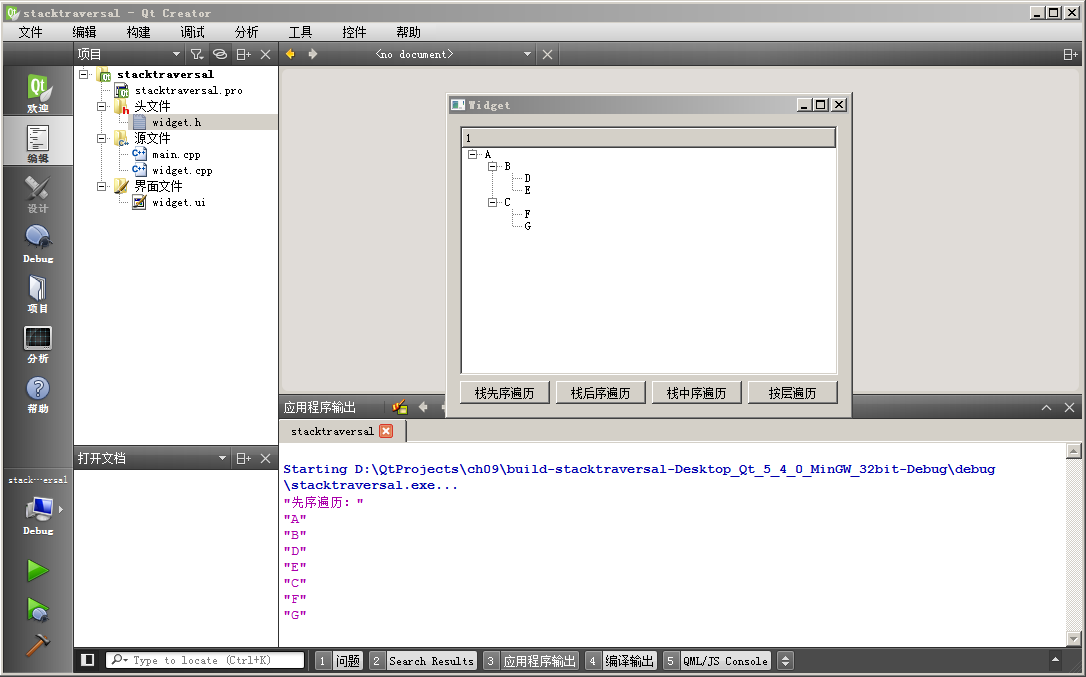9.2.3 顺序容器对比

① 在大多数情况下，QList 都是非常优秀的选择。QList 基于序号的访问机制比链表 QLinkedList 访问效率要高， 通常情况 QList  的访问速度优于 QVector，因为 QList 专门优化过内存存储方式，并且 QList 的代码编写也很简洁。
③ 如果希望元素在内存中连续相邻存储，就是使用封装好的数组，那么 QVector 是适合的选择。
④ Qt 另外提供了低级别的可变长度数组 QVarLengthArray， 比 QVector 函数少一些，QVarLengthArray 不会初始化C++基本类型的元素，而 QVector  总是对元素做初始化。通常情况下，连续空间数组的场景建议优先使用 QVector 。

① 常量时间，记为 O(1) 。即某操作的时间与容器中的元素多少无关，链表的插入操作就是常量时间。
② 对数时间，记为 O(log n)。在容器上的某个操作耗费时间与元素个数的对数成比例关系，对于排序好的数组，使用二分查找 qBinaryFind() 就是对数时间。
③ 线性时间，记为 O(n)。在容器上的某个操作时间直接与元素个数成比例关系，向量的插入操作就是线性时间。
④ 线性对数时间，记为 O(n log n)。在容器上的某个操作时间与（元素个数×元素个数的对数） 成比例关系。快速排序和堆排序的平均算法复杂度就是  O(n log n) 。
⑤ 二次方时间，记为 O(n2) 。在容器上的某个操作的时间与 元素个数的二次方 成比例关系。冒泡排序算法就是二次方时间。

 容器类型 索引查找 随机插入 头部添加 尾部追加 QLinkedList O(n) O(1) O(1) O(1) QList / QQueue O(1) O(n) Amort. O(1) Amort. O(1) QVector / QStack O(1) O(n) O(n) Amort. O(1)

Qt 提供了耗费时间计时器类 QElapsedTimer ，该类的使用举例如下：
QElapsedTimer timer;
timer.start();

slowOperation1();

qDebug() << "The slow operation took" << timer.elapsed() << "milliseconds";

qint64    elapsed() const  //计算耗费的毫秒时间，milliseconds
qint64    nsecsElapsed() const  //计算耗费的纳秒时间，nanoseconds

QLocale alocale = QLocale(QLocale::English, QLocale::UnitedStates);
qDebug()<<alocale.toString( 1234567 );

①项目名称 sequentialtest，创建路径 D:\QtProjects\ch09，点击下一步；
②套件选择里面选择全部套件，点击下一步；
③基类选择 QWidget，点击下一步；
④项目管理不修改，点击完成。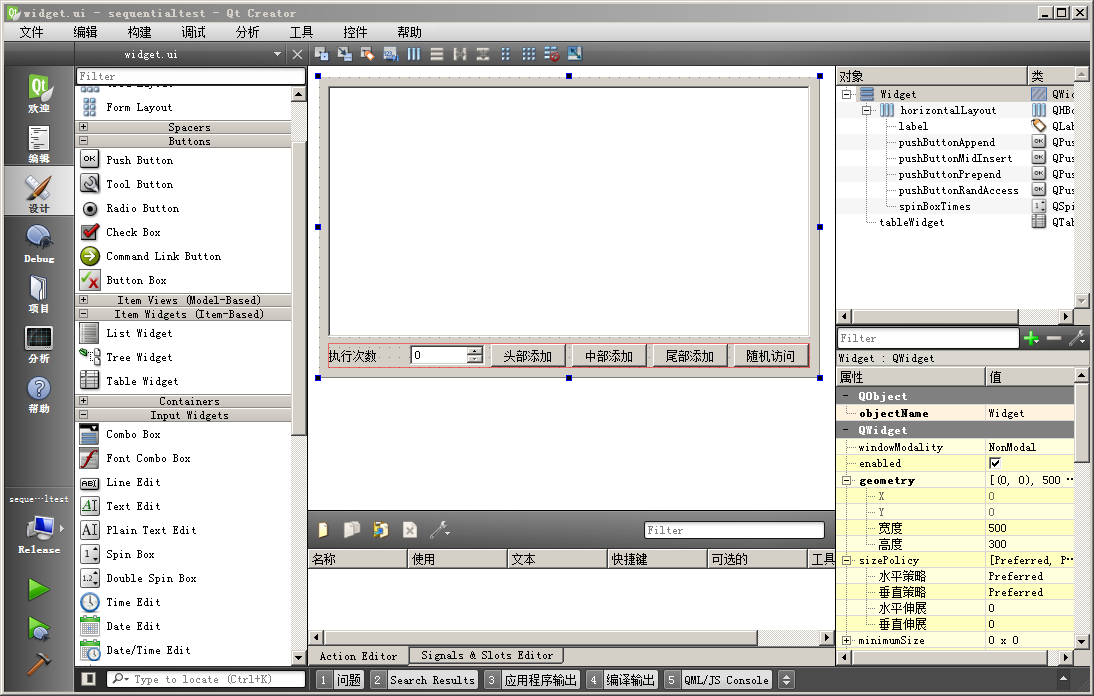#ifndef WIDGET_H
`#define WIDGET_H`

`#include <QWidget>`
`#include <QLinkedList>//链表`
`#include <QList> //列表`
`#include <QVector>//向量`
`#include <QElapsedTimer> //耗费计时`
`#include <QLocale> //本地化类，用于打印逗号分隔的数字`

`namespace Ui {`
`class Widget;`
`}`

`class Widget : public QWidget`
`{`
`    Q_OBJECT`

`public:`
`    explicit Widget(QWidget *parent = 0);`
`    ~Widget();`

`private slots:`
`    void on_pushButtonPrepend_clicked();`

`    void on_pushButtonMidInsert_clicked();`

`    void on_pushButtonAppend_clicked();`

`    void on_pushButtonRandAccess_clicked();`

`private:`
`    Ui::Widget *ui;`
`    //链表`
`    QLinkedList<int> m_linkdList;`
`    //列表`
`    QList<int> m_list;`
`    //向量`
`    QVector<int> m_vector;`
`    //耗费计时器`
`    QElapsedTimer m_calcTimer;`
`    //本地化对象，用于打印逗号分隔的数字`
`    QLocale m_locale;`
`};`

`#endif // WIDGET_H`

`#include "widget.h"`
`#include "ui_widget.h"`
`#include <QDebug>`
`#include <QMessageBox>`
`#include <QTableWidgetItem>//表格控件条目`
`#include <QTableWidget>//表格控件`

`Widget::Widget(QWidget *parent) :`
`    QWidget(parent),`
`    ui(new Ui::Widget)`
`{`
`    ui->setupUi(this);`
`    //设置标题栏`
`    setWindowTitle( tr("顺序容器操作耗时测试，时间单位：纳秒") );`
`    //设置表格列计数`
`    ui->tableWidget->setColumnCount( 4 );`
`    //设置表头`
`    QStringList header;`
`    header<<tr("操作名称")<<tr("QLinkedList")<<tr("QList")<<tr("QVector");`
`    ui->tableWidget->setHorizontalHeaderLabels( header );`
`    //各列均匀拉伸`
`    ui->tableWidget->horizontalHeader()->setSectionResizeMode( QHeaderView::Stretch );`
`    //设置旋钮编辑框`
`    ui->spinBoxTimes->setSuffix( tr(" 万") );//次数的单位是万`
`    ui->spinBoxTimes->setRange( 1, 21*10000 );//测试次数范围从1万到21亿，int上限是 21 亿多`
`    //设置英语本地化，每3个数字使用逗号分隔`
`    m_locale = QLocale(QLocale::English, QLocale::UnitedStates);`
`    qDebug()<<m_locale.toString( 1234567 );`
`}`

`Widget::~Widget()`
`{`
`    delete ui;`
`}`

`//测试头部添加的耗时`
`void Widget::on_pushButtonPrepend_clicked()`
`{`
`    //获取测试次数`
`    int nTimes = ui->spinBoxTimes->value() * 10000;`
`    //表格控件添加新行`
`    int nNewRow = ui->tableWidget->rowCount(); //新增行的序号`
`    ui->tableWidget->setRowCount( nNewRow + 1 );`
`    //设置第 0 列文本`
`    QString strText = tr("头部添加 %1 万次").arg( ui->spinBoxTimes->value() );`
`    QTableWidgetItem *itemNew = new QTableWidgetItem( strText );`
`    ui->tableWidget->setItem(nNewRow, 0, itemNew);`

`    //链表测试，先清空旧的内容`
`    m_linkdList.clear();`
`    qint64 nsUsed = 0;  //统计纳秒数`
`    //开始计时`
`    m_calcTimer.start();`
`    for(int i=0; i<nTimes; i++)`
`    {`
`        m_linkdList.prepend( i ); //头部添加`
`    }`
`    nsUsed = m_calcTimer.nsecsElapsed();//耗时`
`    //设置文本并新建表格条目`
`    strText = m_locale.toString( nsUsed );`
`    itemNew = new QTableWidgetItem(strText);`
`    ui->tableWidget->setItem( nNewRow, 1, itemNew );`

`    //列表测试，先清空旧的内容`
`    m_list.clear();`
`    //开始计时`
`    m_calcTimer.start();`
`    //提前保留元素空间，避免频繁分配空间和复制元素`
`    m_list.reserve( nTimes );`
`    for(int i=0; i<nTimes; i++)`
`    {`
`        m_list.prepend( i );    //头部添加`
`    }`
`    nsUsed = m_calcTimer.nsecsElapsed();//耗时`
`    //设置文本并新建表格条目`
`    strText = m_locale.toString( nsUsed );`
`    itemNew = new QTableWidgetItem(strText);`
`    ui->tableWidget->setItem( nNewRow, 2, itemNew );`

`    //向量测试，先清空旧的`
`    m_vector.clear();`
`    //开始计时`
`    m_calcTimer.start();`
`    //提前保留元素空间`
`    m_vector.reserve( nTimes );`
`    for(int i=0; i<nTimes; i++)`
`    {`
`        m_vector.prepend( i );  //头部添加`
`    }`
`    nsUsed = m_calcTimer.nsecsElapsed();//耗时`
`    //设置文本并新建表格条目`
`    strText = m_locale.toString( nsUsed );`
`    itemNew = new QTableWidgetItem(strText);`
`    ui->tableWidget->setItem( nNewRow, 3, itemNew );`

`    //滚动到最下面新建行`
`    ui->tableWidget->scrollToBottom();`
`}`

`//测试中部添加的耗时`
`void Widget::on_pushButtonMidInsert_clicked()`
`{`
`    int nTimes = ui->spinBoxTimes->value() * 10000;`
`    //表格控件添加新行`
`    int nNewRow = ui->tableWidget->rowCount(); //新增行的序号`
`    ui->tableWidget->setRowCount( nNewRow + 1 );`
`    //设置第 0 列文本`
`    QString strText = tr("中部添加 %1 万次").arg( ui->spinBoxTimes->value() );`
`    QTableWidgetItem *itemNew = new QTableWidgetItem( strText );`
`    ui->tableWidget->setItem(nNewRow, 0, itemNew);`

`    //链表测试，先清空旧的内容`
`    m_linkdList.clear();`
`    qint64 nsUsed = 0;  //统计纳秒数`
`    QLinkedList<int>::iterator it; //迭代器`
`    //开始计时`
`    m_calcTimer.start();`
`    for(int i=0; i<nTimes; i++)`
`    {`
`        int nMid = m_linkdList.count() / 2;`
`        //用迭代器访问，链表如果知道指向元素的迭代器，那么增删效率高`
`        //如果不知道中间元素迭代器，那么迭代器遍历的时间比较长`
`        it = m_linkdList.begin();`
`        for(int j=0; j<nMid; j++)`
`        {`
`            it++;`
`        }`
`        //中部添加`
`        m_linkdList.insert(it, i);`
`    }`
`    nsUsed = m_calcTimer.nsecsElapsed();//耗时`
`    //设置文本并新建表格条目`
`    strText = m_locale.toString( nsUsed );`
`    itemNew = new QTableWidgetItem(strText);`
`    ui->tableWidget->setItem( nNewRow, 1, itemNew );`

`    //列表测试，先清空旧的`
`    m_list.clear();`
`    //开始计时`
`    m_calcTimer.start();`
`    //提前保留元素空间，避免频繁分配空间和复制元素`
`    m_list.reserve( nTimes );`
`    for(int i=0; i<nTimes; i++)`
`    {`
`        m_list.insert( m_list.count()/2, i );`
`    }`
`    nsUsed = m_calcTimer.nsecsElapsed();//耗时`
`    //设置文本并新建表格条目`
`    strText = m_locale.toString( nsUsed );`
`    itemNew = new QTableWidgetItem(strText);`
`    ui->tableWidget->setItem( nNewRow, 2, itemNew );`

`    //向量测试，先清空旧的`
`    m_vector.clear();`
`    //开始计时`
`    m_calcTimer.start();`
`    //提前保留元素空间`
`    m_vector.reserve( nTimes );`
`    for(int i=0; i<nTimes; i++)`
`    {`
`        m_vector.insert( m_vector.count()/2, i );`
`    }`
`    nsUsed = m_calcTimer.nsecsElapsed();//耗时`
`    //设置文本并新建表格条目`
`    strText = m_locale.toString( nsUsed );`
`    itemNew = new QTableWidgetItem(strText);`
`    ui->tableWidget->setItem( nNewRow, 3, itemNew );`

`    //滚动到最下面新建行`
`    ui->tableWidget->scrollToBottom();`
`}`

计算中部的序号 nMid；
获取链表开始的迭代器，用内层循环遍历迭代器 nMid-1 次，这时迭代器正好指向序号为 nMid 的元素；
在中部元素之前插入新元素，数值为  i。

`//测试尾部添加的耗时`
`void Widget::on_pushButtonAppend_clicked()`
`{`
`    //获取测试次数`
`    int nTimes = ui->spinBoxTimes->value() * 10000;`
`    //表格控件添加新行`
`    int nNewRow = ui->tableWidget->rowCount(); //新增行的序号`
`    ui->tableWidget->setRowCount( nNewRow + 1 );`
`    //设置第 0 列文本`
`    QString strText = tr("尾部添加 %1 万次").arg( ui->spinBoxTimes->value() );`
`    QTableWidgetItem *itemNew = new QTableWidgetItem( strText );`
`    ui->tableWidget->setItem(nNewRow, 0, itemNew);`

`    //链表测试，先清空旧的`
`    m_linkdList.clear();`
`    qint64 nsUsed = 0;  //统计纳秒数`
`    //开始计时`
`    m_calcTimer.start();`
`    for(int i=0; i<nTimes; i++)`
`    {`
`        m_linkdList.append( i );//尾部添加`
`    }`
`    nsUsed = m_calcTimer.nsecsElapsed();//耗时`
`    //设置文本并新建表格条目`
`    strText = m_locale.toString( nsUsed );`
`    itemNew = new QTableWidgetItem(strText);`
`    ui->tableWidget->setItem( nNewRow, 1, itemNew );`

`    //列表测试，先清空旧的`
`    m_list.clear();`
`    //开始计时`
`    m_calcTimer.start();`
`    //提前保留元素空间`
`    m_list.reserve( nTimes );`
`    for(int i=0; i<nTimes; i++)`
`    {`
`        m_list.append( i );//尾部添加`
`    }`
`    nsUsed = m_calcTimer.nsecsElapsed();//耗时`
`    //设置文本并新建表格条目`
`    strText = m_locale.toString( nsUsed );`
`    itemNew = new QTableWidgetItem(strText);`
`    ui->tableWidget->setItem( nNewRow, 2, itemNew );`

`    //向量测试，先清空旧的`
`    m_vector.clear();`
`    //开始计时`
`    m_calcTimer.start();`
`    //提前保留元素空间`
`    m_vector.reserve( nTimes );`
`    for(int i=0; i<nTimes; i++)`
`    {`
`        m_vector.append( i );//尾部添加`
`    }`
`    nsUsed = m_calcTimer.nsecsElapsed();//耗时`
`    //设置文本并新建表格条目`
`    strText = m_locale.toString( nsUsed );`
`    itemNew = new QTableWidgetItem(strText);`
`    ui->tableWidget->setItem( nNewRow, 3, itemNew );`

`    //滚动到最下面新建行`
`    ui->tableWidget->scrollToBottom();`
`}`
“尾部添加”按钮的槽函数与“头部添加”按钮的槽函数内容几乎一样，仅仅是将容器的 prepend() 函数换成了 append() 函数，这里就不赘述了。

`void Widget::on_pushButtonRandAccess_clicked()`
`{`
`    //获取测试次数`
`    int nTimes = ui->spinBoxTimes->value() * 10000;`
`    //查看元素数量是否有 nTimes 个`
`    if( m_list.count() < nTimes )`
`    {`
`        QMessageBox::warning(this, tr("随机访问"), tr("请先进行任意其他项测试为顺序容器添加元素。"));`
`        return;`
`    }`
`    //每个容器已经有了 nTimes 个元素`
`    //表格控件添加新行`
`    int nNewRow = ui->tableWidget->rowCount(); //新增行的序号`
`    ui->tableWidget->setRowCount( nNewRow + 1 );`
`    //设置第 0 列文本`
`    QString strText = tr("随机访问 %1 万次").arg( ui->spinBoxTimes->value() );`
`    QTableWidgetItem *itemNew = new QTableWidgetItem( strText );`
`    ui->tableWidget->setItem(nNewRow, 0, itemNew);`
`    //计时变量和随机序号变量`
`    qint64 nsUsed = 0; //纳秒计时`
`    int nIndex = 0; //随机序号`

`    //链表测试，随机访问`
`    int nAllCount = m_linkdList.count(); //元素数量`
`    //开始计时`
`    m_calcTimer.start();`
`    for(int i=0; i<nTimes; i++)`
`    {`
`        //生成新的随机序号`
`        nIndex = ( nIndex + qrand() ) % nAllCount;`
`        //迭代器`
`        QLinkedList<int>::iterator it = m_linkdList.begin();`
`        for(int j=0; j<nIndex; j++) //只能用迭代器查找元素`
`        {`
`            it++;`
`        }`
`        (*it) += i; //获取元素值并加上 i`
`    }`
`    nsUsed = m_calcTimer.nsecsElapsed();//耗时`
`    //设置文本并新建表格条目`
`    strText = m_locale.toString( nsUsed );`
`    itemNew = new QTableWidgetItem(strText);`
`    ui->tableWidget->setItem( nNewRow, 1, itemNew );`

`    //列表随机访问测试`
`    nAllCount = m_list.count(); //元素的数量`
`    //开始计时`
`    m_calcTimer.start();`
`    for(int i=0; i<nTimes; i++)`
`    {`
`        //生成新的随机序号`
`        nIndex = ( nIndex + qrand() ) % nAllCount;`
`        m_list[ nIndex ] += i; //将元素值加 i`
`    }`
`    nsUsed = m_calcTimer.nsecsElapsed();//耗时`
`    //设置文本并新建表格条目`
`    strText = m_locale.toString( nsUsed );`
`    itemNew = new QTableWidgetItem(strText);`
`    ui->tableWidget->setItem( nNewRow, 2, itemNew );`

`    //向量随机访问测试`
`    nAllCount = m_vector.count();`
`    //开始计时`
`    m_calcTimer.start();`
`    for(int i=0; i<nTimes; i++)`
`    {`
`        //生成新的随机序号`
`        nIndex = ( nIndex + qrand() ) % nAllCount;`
`        m_vector[ nIndex ] += i; //将元素值加 i`
`    }`
`    nsUsed = m_calcTimer.nsecsElapsed();//耗时`
`    //设置文本并新建表格条目`
`    strText = m_locale.toString( nsUsed );`
`    itemNew = new QTableWidgetItem(strText);`
`    ui->tableWidget->setItem( nNewRow, 3, itemNew );`

`    //滚动到最下面新建行`
`    ui->tableWidget->scrollToBottom();`
`}`

设置新的随机序号 nIndex，计算方式是旧的序号加新随机数，然后模 nAllCount ；
定义迭代器，获取列表的开始迭代器；
用内层循环遍历迭代器 nIndex -1 次，这样迭代器 it 正好指向序号为 nIndex 的元素；
将 nIndex 序号的元素数值加 i 。